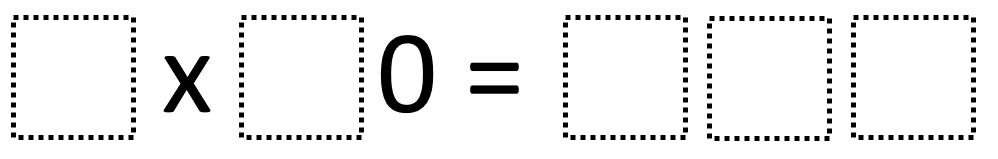# Multiplying Multiples Of Ten 1

Directions: Using the digits 0 to 9 at most one time each, place a digit in each box to make two different true number sentences: one with a product that’s less than 500 and one with a product that’s greater than 500. You may reuse all the digits each product.### Hint

How can you tell where the zero will go? How can your knowledge of multiplying one-digit numbers help you here?

There are many possible answers including 9 x 30 = 270 and 8 x 70 = 560

Source: Robert Kaplinsky

## Multiply and Divide Within A Hundred 1

Directions: Using the digits 2 to 9 at most one time each, place a digit …

1.2.3.